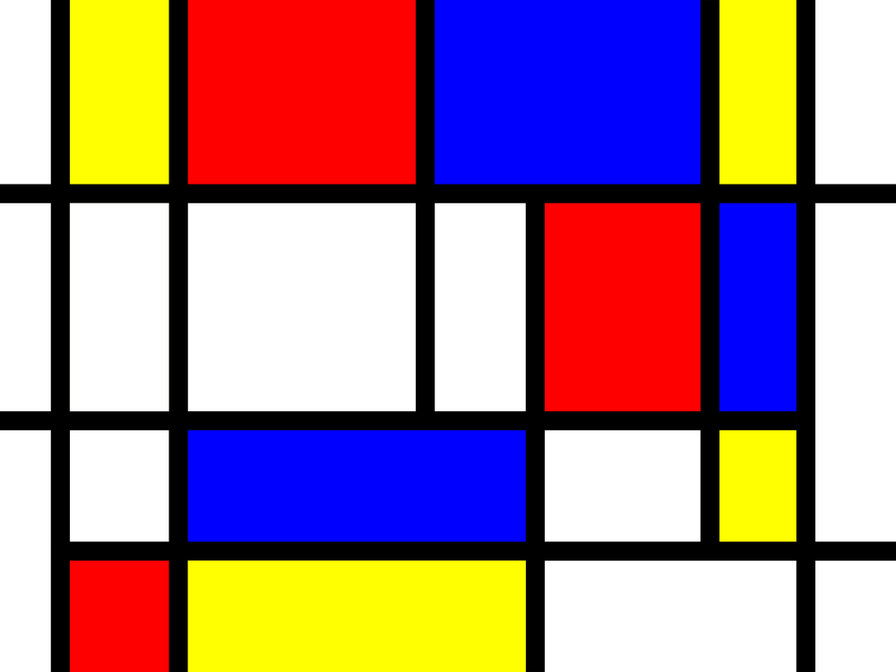### Conformal Prediction

How good is your prediction? If you are predicting the label of a new object, how confident are you that the predicted label is correct? If the label is a number, how close it is to the correct one?

In machine learning, these questions are often answered in a fairly rough way. CP uses past experience to determine precise confidence levels in predictions. The Piet Mondrian paintings inspired for Mondrian CPs where different error rates are for different classes.### Support Vector Machine

Classifying data is a common task in machine learning. Suppose some given data points each belong to one of two classes, and the goal is to decide which class a new Data point will be in.

In the case of SVM, a data point is viewed as a list of p numbers, and we want to know if they can be separated by a (p-1)-dimensional hyperplane, so that the distance from it to the nearest data point on each side is maximised.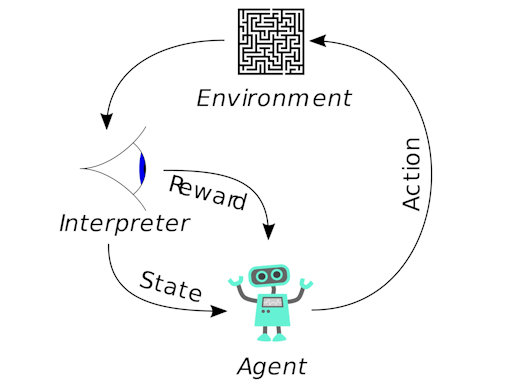### Q-Learning

Q-Learning is a family of Reinforcement Learning. The idea of Q-Learning is that we have a representation of the environmental states, and the possible actions for those states. Afterwards, we learn the value of the action in each state.

We first set all state-action values to 0, then go around and explore the state-action space. After trying an action, if it leads to an undesirable outcome, we will reduce the weight of that action from that state, so that other actions will be chosen instead the next time we are in that state.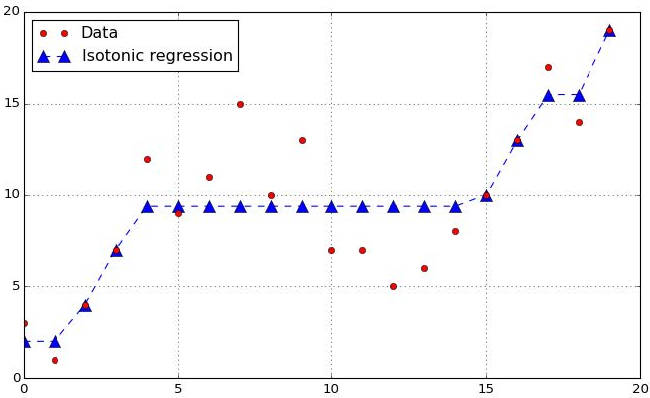### Venn-Abers

Venn predictors produce probability-type predictions for the labels of test objects which are guaranteed to be well calibrated under the standard assumption that the observations are generated independently from the same distribution.

Many machine learning algorithms for classification are scoring classifiers: they output a prediction score s(x) and the prediction is obtained by comparing the score to a threshold. One could apply a function g to s(x) to calibrate the scores so that g(s(x)) can be used as predicted probability.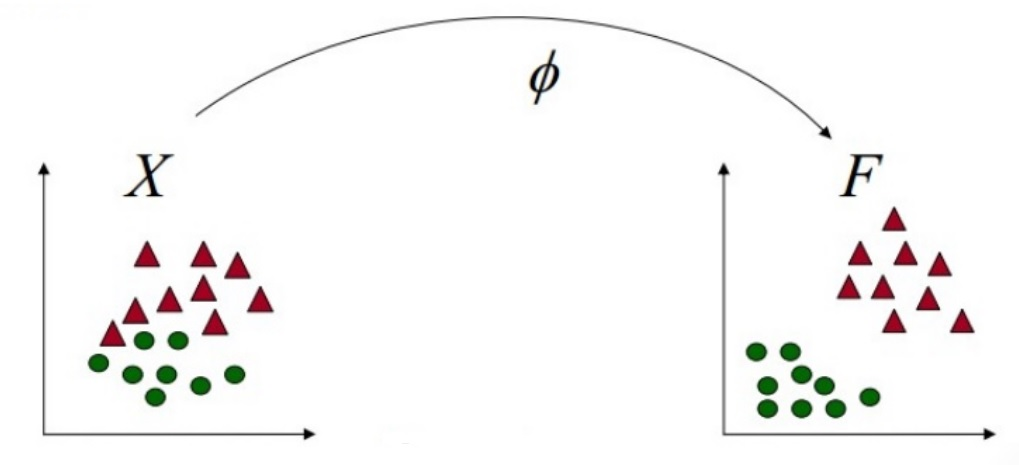### String kernels

Suppose one wants to compare some text passages automatically and indicate their relative similarity. One example where exact matching is not always enough is found in spam detection. Another would be in computational gene analysis, where homologous genes have mutated.

A string kernel is a kernel function that operates on strings. String kernels can be intuitively understood as functions measuring the similarity of pairs of strings: the more similar two strings a and b are, the higher the value of a string kernel K(a, b) will be. Using string kernels with kernelized learning algorithms such as SVM allow such algorithms to work with strings.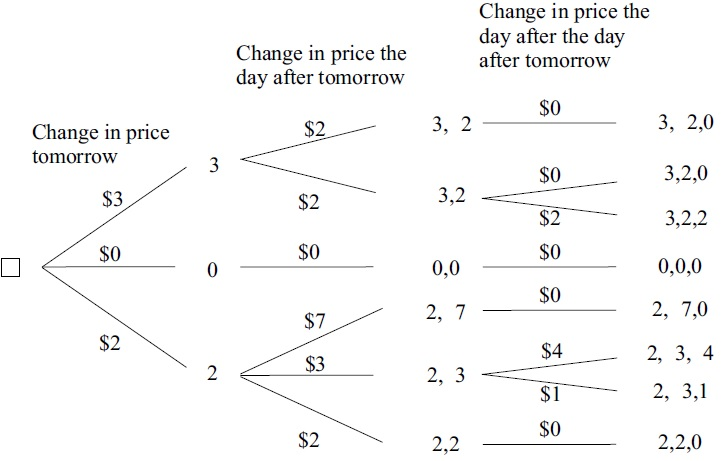### Game-Theoretic Probability

We proposed a framework for the theory and use of mathematical probability that rests more on game theory than on measure theory. This new framework merits attention on purely mathematical grounds, it captures the basic intuitions of probability simply and effectively.

Our framework is a straightforward but rigorous elaboration, with no extraneous mathematical or philosophical baggage, of two ideas that are fundamental to both probability and finance: The Principle of Pricing by Dynamic Hedging, and The Hypothesis of the Impossibility of a Gambling System.### Online compression model

An online compression model (OCM) is an automaton for summarising statistical information efficiently. It is usually impossible to restore the statistical information from the OCM's summary, but it can be argued that the only information lost is noise, since one of our requirements is that the summary should be a "sufficient statistic".

OCM a step towards implementation of Kolmogorov's program for applications of probability; in particular, the concept of OCM is an on-line version of the concept considered by Kolmogorov.